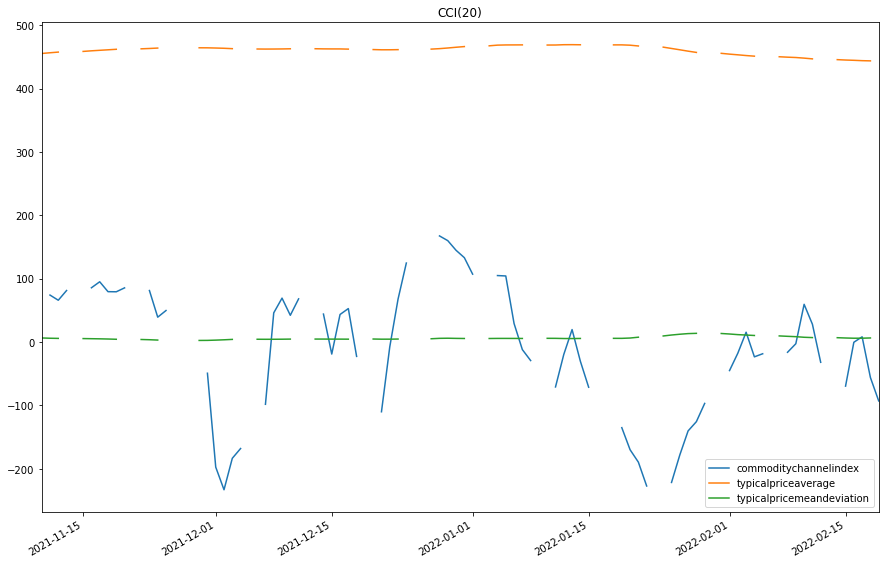# Indicator Reference

## Commodity Channel Index

### Introduction

This indicator represents the traditional commodity channel index (CCI) CCI = (Typical Price - 20-period SMA of TP) / (.015 * Mean Deviation) Typical Price (TP) = (High + Low + Close)/3 Constant = 0.015 There are four steps to calculating the Mean Deviation, first, subtract the most recent 20-period average of the typical price from each period's typical price. Second, take the absolute values of these numbers. Third, sum the absolute values. Fourth, divide by the total number of periods (20).

### Create Manual Indicators

You can manually create a CommodityChannelIndex indicator, so it doesn’t automatically update. Manual indicators let you update their values with any data you choose. The following reference table describes the CommodityChannelIndex constructor.

### CommodityChannelIndex()1/2

            CommodityChannelIndex QuantConnect.Indicators.CommodityChannelIndex (
int                 period,
*MovingAverageType  movingAverageType
)


Initializes a new instance of the CommodityChannelIndex class.

### CommodityChannelIndex()2/2

            CommodityChannelIndex QuantConnect.Indicators.CommodityChannelIndex (
string              name,
int                 period,
*MovingAverageType  movingAverageType
)


Initializes a new instance of the CommodityChannelIndex class.

The following table shows the MovingAverageType enumeration members and the indicator that implements each one:

MovingAverageTypeUnderlying Indicator
SimpleSimple Moving Average
ExponentialExponential Moving Average
WildersWilder Moving Average
LinearWeightedMovingAverageLinear Weighted Moving Average
DoubleExponentialDouble Exponential Moving Average
TripleExponentialTriple Exponential Moving Average
TriangularTriangular Moving Average
T3T3 Moving Average
KamaKaufman Adaptive Moving Average
HullHull Moving Average
AlmaArnaud Legoux Moving Average

### Update Manual Indicators

You can update the indicator automatically or manually.

#### Automatic Update

To register a manual indicator for automatic updates with the security data, call the RegisterIndicator method.


private CommodityChannelIndex _cci;

// In Initialize()
_cci = new CommodityChannelIndex(name, period);
_cci.Updated += IndicatorUpdateMethod;

RegisterIndicator(symbol, _cci, Resolution.Daily);

// In IndicatorUpdateMethod()
{
var indicatorValue = _cci.Current.Value;
}

# In Initialize()
self.cci = CommodityChannelIndex(name, period)
self.cci.Updated += self.IndicatorUpdateMethod

self.RegisterIndicator(symbol, self.cci, Resolution.Daily)

# In IndicatorUpdateMethod()
indicator_value = self.cci.Current.Value

To customize the data that automatically updates the indicator, see Custom Indicator Periods and Custom Indicator Values.

#### Manual Update

Updating your indicator manually enables you to control when the indicator is updated and what data you use to update it. To manually update the indicator, call the Update method with a TradeBar, QuoteBar, or an IndicatorDataPoint. The indicator will only be ready after you prime it with enough data.


private CommodityChannelIndex _cci;
private Symbol symbol;

// In Initialize()
_cci = new CommodityChannelIndex(period);

// In OnData()
if (data.QuoteBars.ContainsKey(_symbol))
{
_cci.Update(data.QuoteBars[symbol]);
}
{
var indicatorValue = _cci.Current.Value;
}

# In Initialize()
self.cci = CommodityChannelIndex(period)

# In OnData()
if data.QuoteBars.ContainsKey(self.symbol):
self.cci.Update(data.QuoteBars[self.symbol])
indicator_value = self.cci.Current.Value

### Create Automatic Indicators

The CCI method creates an CommodityChannelIndex indicator, sets up a consolidator to update the indicator, and then returns the indicator so you can use it in your algorithm.

The following reference table describes the CCI method:

### CCI()1/1

            CommodityChannelIndex QuantConnect.Algorithm.QCAlgorithm.CCI (
Symbol                                symbol,
Int32                                 period,
*MovingAverageType                    movingAverageType,
*Nullable<Resolution>           resolution,
*Func<IBaseData, IBaseDataBar>  selector
)


Creates a new CommodityChannelIndex indicator. The indicator will be automatically updated on the given resolution.

The following table shows the MovingAverageType enumeration members and the indicator that implements each one:

MovingAverageTypeUnderlying Indicator
SimpleSimple Moving Average
ExponentialExponential Moving Average
WildersWilder Moving Average
LinearWeightedMovingAverageLinear Weighted Moving Average
DoubleExponentialDouble Exponential Moving Average
TripleExponentialTriple Exponential Moving Average
TriangularTriangular Moving Average
T3T3 Moving Average
KamaKaufman Adaptive Moving Average
HullHull Moving Average
AlmaArnaud Legoux Moving Average

If you don't provide a resolution, it defauls to the security resolution. If you provide a resolution, it must be greater than or equal to the resolution of the security. For instance, if you subscribe to hourly data for a security, you should update its indicator with data that spans 1 hour or longer.

### Get Indicator Values

To get the value of the indicator, use its Current.Value attribute.

private CommodityChannelIndex _cci;

// In Initialize()
_cci = CCI(symbol, period);

// In OnData()
{
var typicalPriceAverage = _cci.TypicalPriceAverage.Current.Value;
var typicalPriceMeanDeviation = _cci.TypicalPriceMeanDeviation.Current.Value;
var current = _cci.Current.Value;
}
# In Initialize()
self.cci = self.CCI(symbol, period)

# In OnData()
typical_price_average = self.cci.TypicalPriceAverage.Current.Value
typical_price_mean_deviation = self.cci.TypicalPriceMeanDeviation.Current.Value
current = self.cci.Current.Value


### Visualization

To plot indicator values, in the OnData event handler, call the Plot method.

private CommodityChannelIndex _cci;

// In Initialize()
_cci = CCI(symbol, period);

// In OnData()
{
Plot("My Indicators", "typicalpriceaverage", _cci.TypicalPriceAverage);
Plot("My Indicators", "typicalpricemeandeviation", _cci.TypicalPriceMeanDeviation);
Plot("My Indicators", "commoditychannelindex", _cci.Current);
}
# In Initialize()
self.cci = self.CCI(symbol, period)

# In OnData()